﻿ Einstein's Mass-Energy EquivalenceMost of these introductory articles are exracted from Volume I of the Single Monad Model of the Cosmos: Ibn al-Arabi's View of Time and Creation... more on this can be found here.

## Einstein's Mass-Energy Equivalence

Note that if we want to consider mass to be variable with speed and distinguish between rest mass: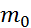, and relativistic mass: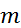, according to the standard equation: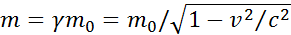(4)

Then we can arrive to Einstein’s equation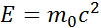by calculating the derivative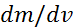which in this case will not be equal to zero. However, the above relativistic mass in equation number (4) is only obtained based on the same Einstein’s equationthat we are trying to prove here, so in this case it will be a circular argument. Actually there are various other methods to prove this equation, but all has been criticized because they are either applicable only in certain situations or rely on some relativistic concepts. Apart from the overwhelming experimental confirmation, neither Einstein, nor anyone else, had ever been able to construct a comprehensive prove for this famous relation from first fundamental principles [].

The only way to prove this relation classically is to suppose that velocity jumps from zero to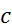in “no time”, and that is exactly what the Re-creation Principle is postulating. In this case the above integration in equation (2) will be converted back into summation of the two discrete states of zero and, because in calculus integration and derivation are only valid with infinitesimal change: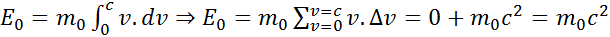(5)

The difference between these two cases that result in equations (3) and (5) is demonstrated in Figure 1 below.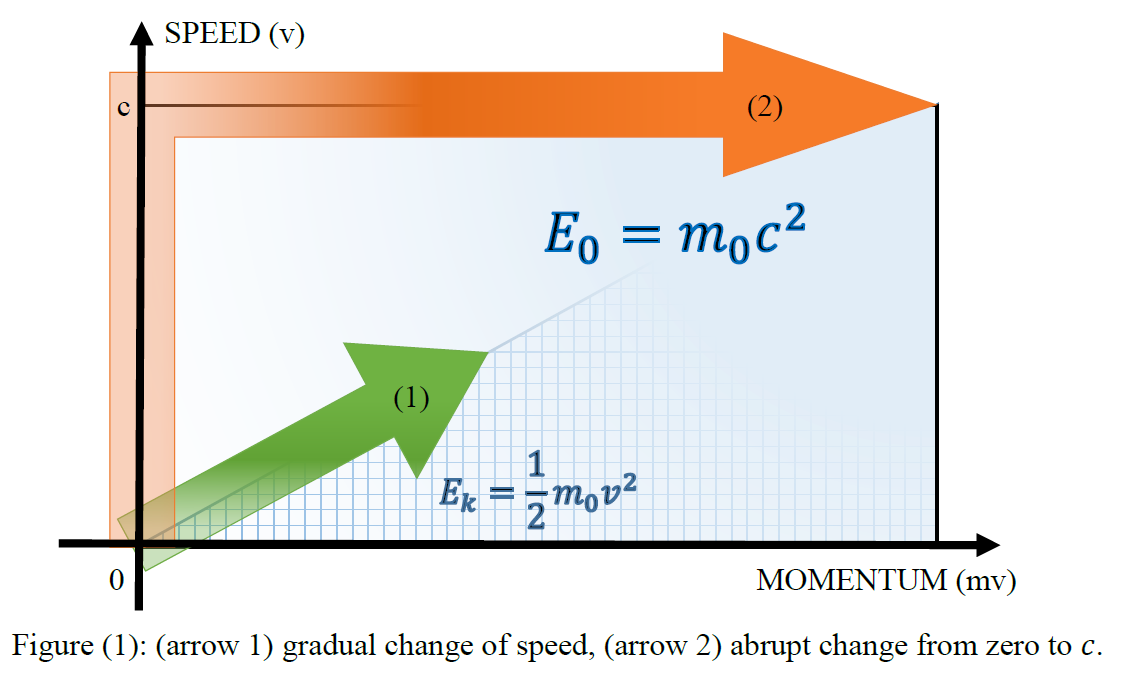Figure (1):

The difference between gradual change of speed and abrupt change.

So this would be a straightforward mathematical proof of the Re-Creation Principle, but we only need first to physically justify this abrupt change in velocity. Obviously, the problem is that with zero-time intervals, the acceleration would be infinite, and hence the force and energy. Light does in fact move from zero toand vice versa, for example when emitted or absorbed, but the photon is massless, unlike other particles and objects which have mass and suffer inertia and acceleration. In fact, we are now faced with the same dilemma of the wave-particle duality, or continuity versus discreteness, and as we said above, the only way out of this dilemma is “oneness”.

We shall see in the following [section ?6] that on the primary level of oneness everything happens in “no time”, because the simple monads are naturally massless, so the task would be the other way around by explaining how massive particles and objects move with limited velocities on the normal level of space and time, or how do they acquire mass, or equally: how monads are coupled together to construct space-time itself, where multiplicity is hosted.

We can alternatively try to explain this zero-time interval in terms of quantum tunneling which has been reported to have occurred in zero-time [] or superluminally [], but since we are trying here to explain the reality, we want to discuss the general philosophical reasons that make all this possible. Once these reasons are clarified, zero-time tunneling and other nonlocal quantum phenomena would also be explained even outside the mathematical formulation of Quantum Mechanics, that would also gain stronger supporting logic.

When “particles” appear to maintain their motion at the speed of light, they are said to be massless, such as the photon for example, because its rest mass has been completely converted into energy. So this mass-energy equivalence is the usual operation that is always happening at the ontological level, as a result of exciting the vacuum field into waves and their eventual collapsing back into vacuum, which is the described perpetual alternation of monads between existence and nonexistence, that is always conveyed by the Single Monad.

On the other hand, on the phenomenological level, when during a time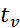a monad or an object is moving at an average limited velocity that is a result of averaging the dual-state velocity or the average of various vector velocities of its elementary particles that are moving in all directions, so on average it is spending some time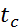moving at(that corresponds to existence) and some time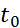at zero (that corresponds to rest, nonexistence or vacuum), then in total it may move a distance: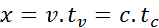(6)

Because it performed no distance during. Therefore, this average velocity is given by: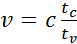(7)

And its kinetic energy will be given by same standard classical equation (3):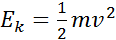, because its change is smooth.

However, since this average velocity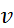is the apparent velocity that is a “mixture” of its instantaneous dual-state velocity (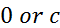), whenever this object or monad is measured or detected it will be necessarily at rest, at the real instance of measurement in the real flow of time, with its initial constant rest mass, as a result of the collapse of wave function. This is in fact the philosophical reason behind the first principle of Special Relativity, i.e. the laws of physics are invariant in all inertial systems that are non-accelerating frames of reference, because there is no difference at all if the monad or object is at rest or moving with any constant speed, since in reality it is always at rest at the time of measurement. This also means that even when it is moving at any speed that could be very close to c, at the time of measurement its mass will still be the same rest mass, because it is only detected as a particle, while its energy will be given by its relativistic mass, and then its total energy equivalence, with relation to an observer moving at a constant velocity, will be given by: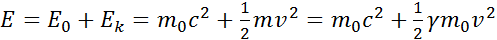(8)

This is the only philosophical explanation that can account for the existence of “rest mass” and “relativistic mass” in the same equation. Mass itself is always the rest mass, it is not relativistic, but its effect, i.e. energy is.

Therefore, in conclusion, this notion of re-creation, or dual-state velocity, is able to explain at once all the three postulates of Special Relativity: the mass-energy equivalence, the invariance of physics laws in inertial frames, and the constancy of the speed of light, in addition to explaining the nature and collapse of the wave function into eigenstate. In a coming paper, we want to explore how we can apply this principle on General Relativity.

 Leaving aside that it continues to be affirmed experimentally, a rigorous proof of the mass-energy equivalence is probably beyond the purview of the special theory: Hecht, Eugene (2011), "How Einstein confirmed E0=mc2", American Journal of Physics, 79 (6): 591–600, Bibcode:2011AmJPh..79..591H, doi:10.1119/1.3549223.

 Low, F. E. (1998). "Comments on apparent superluminal propagation". Ann. Phys. Leipzig. 7 (7–8): 660–661. Bibcode:1998AnP...510..660L. doi:10.1002/(SICI)1521-3889(199812)7:7/8<660::AID-ANDP660>3.0.CO;2-0. Nimtz, G. (2011). "Tunneling Confronts Special Relativity". Found. Phys. 41 (7): 1193–1199. arXiv:1003.3944free to read. Bibcode:2011FoPh...41.1193N. doi:10.1007/s10701-011-9539-2.

 Razavy, Mohsen (2003). Quantum Theory of Tunneling. World Scientific. pp. 4, 462. ISBN 9812564888.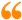The science of Time is a noble science, that reveals the secret of Eternity. Only the Elites of Sages may ever come to know this secret. It is called the First Age, or the Age of ages, from which time is emerging.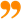Ibn al-Arabi [The Meccan Revelations: Volume I, page 156. - Trns. Mohamed Haj Yousef]

### Ibn al-Arabi Website:### The Meccan Revelations:### The Sun from the West:### Message from the Author:I have no doubt that this is the most significant discovery in the history of mathematics, physics and philosophy, ever!

This unique understanding of geometry will cause a paradigm shift in our knowledge of the fundamental nature of the cosmos and its corporeal and incorporeal structures.

Enjoy reading... , all the Best !

Mohamed bin Ali Haj Yousef

Check this detailed video presentation on "Deriving the Principles of Special, General and Quantum Relativity Based on the Single Monad Model Cosmos and Duality of Time Theory".

## Social SharingAllah is Beautiful, and He loves beauty.